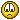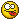## HELP!! Tracking, Connecting to Tracker And Garbage matte on Ultra Keyer

Moderator: SecondMan

Ayush Bakshi
Posts: 15
Joined: Wed Jan 23, 2019 8:59 am
Been thanked: 2 times

### HELP!! Tracking, Connecting to Tracker And Garbage matte on Ultra Keyer

It's draining my energy (and possibly sanity) but I can't figure out: (Images attached down there ↓)

1. How to use garbage matte with Ultra Keyer?
I attached B-spline's node to Ultra Keyer's 'Garbage Matte'. It worked half expectedly (literally). It didn't performed keying operation in the garbage mask area (as expected) but it didn't masked the entire thing... I just wanted that part masked outIt did worked as expected when I check 'Post multiply image' but it gave black edges.
Also, It (spline node connected to 'Garbage Matte') behaved normally with Delta keyer.

2. How to connect splines' position to tracker without moving the spline?
I set spline → Happy with the shape and position → Tracked something using 'Tracker' node → Connected spline's 'Center' to tracker's 'Offset position' (right decision?). The problem is that this operation moved the spline which I don't want. I can reposition it by selecting all the anchor points but it's not easy to redo when the shape is complex and you already spent time in positioning every single point.
Also what's the difference between all those 'connect to' options (refer to attached images).

What am I doing wrong? Just want to keep my life simple but Fusion is trickier than I anticipated for :'(
There are so few tutorials for BMD Fusion (comparatively speaking). In future I'll be using Nuke because BMD fusion isn't used much in Industries (in my country). But I've to use it because it's in my course [crying with tears emoji]

Images↓

It also crashes randomly in an instant but that's the problem for another thread...

Movalex
Fusioneer
Posts: 94
Joined: Fri Nov 03, 2017 5:36 am
Been thanked: 20 times
Contact:

### Re: HELP!! Tracking, Connecting to Tracker And Garbage matte on Ultra Keyer

1. Uncheck Subtract background when premultiplying Ultrakeyer or you'll have double premultiplication. Also try Deltakeyer, I like it better.
2. Tracker Plus from Reactor has Set/Change reference time setting. Probably it could help.

UPD:
a) install Eyeon Legacy scripts from Reactor.
b) select tracker on the frame you need
c) add spline on the same frame and right click - Script- Eyeon Legacy -- Attach Mask... and choose current Tracker Path
You do not have the required permissions to view the files attached to this post.

Ayush Bakshi
Posts: 15
Joined: Wed Jan 23, 2019 8:59 am
Been thanked: 2 times

### Re: HELP!! Tracking, Connecting to Tracker And Garbage matte on Ultra Keyer

Movalex wrote:
Wed Feb 13, 2019 2:54 am
a) install Eyeon Legacy scripts from Reactor.
b) select tracker on the frame you need
c) add spline on the same frame and right click - Script- Eyeon Legacy -- Attach Mask... and choose current Tracker Path
Thanks! Ultra Keyer Problem Solved.

I did what you said and applied that script to Transform node. It worked. 7 Splines are connected in chain and on the end is Transform node. Splines are moving with it (as expected) BUT.. When I connected 'Transform node OUT' to Delta Keyer's Solid matte... It works only for first frame.. After that splines doesn't affect Solid matte as if they aren't there. If I don't introduce Transform node then things work fine.

Image ↓

Movalex
Fusioneer
Posts: 94
Joined: Fri Nov 03, 2017 5:36 am
Been thanked: 20 times
Contact:

### Re: HELP!! Tracking, Connecting to Tracker And Garbage matte on Ultra Keyer

Did you try Flatten Transform? Also check if it is a memory issue (purge cache)

Ayush Bakshi
Posts: 15
Joined: Wed Jan 23, 2019 8:59 am
Been thanked: 2 times

### Re: HELP!! Tracking, Connecting to Tracker And Garbage matte on Ultra Keyer

Movalex wrote:
Wed Feb 13, 2019 6:21 am
Did you try Flatten Transform? Also check if it is a memory issue (purge cache)
I tried both. Nothing changed.
If someone want to achieve this then is it the right approach? Logically speaking... I can't understand why it's not working.

SirEdric
Fusionator
Posts: 1753
Joined: Tue Aug 05, 2014 10:04 am
Real name: Eric Westphal
Been thanked: 80 times
Contact:

### Re: HELP!! Tracking, Connecting to Tracker And Garbage matte on Ultra Keyer

So you're piping the RGB out of the Tracker into the Garbagemask input?
While in theory this should work, what happens if you add a BitmapMask to the GarbageInout first and pipe the XF into BitmapMask?

Added in 3 minutes 5 seconds:
And/or (just to be ultra-safe to not mix up RGB/Mask pipes) something like this:

Code: Select all

{
Tools = ordered() {
DrawMode = "InsertAndModify",
DrawMode2 = "InsertAndModify",
Inputs = {
MaskWidth = Input { Value = 1920, },
MaskHeight = Input { Value = 1080, },
PixelAspect = Input { Value = { 1, 1 }, },
ClippingMode = Input { Value = FuID { "None" }, },
Polyline = Input {
SourceOp = "Polygon1Polyline",
Source = "Value",
},
Polyline2 = Input {
Value = Polyline {
},
Disabled = true,
},
},
ViewInfo = OperatorInfo { Pos = { 55, -16.5 } },
},
Polygon1Polyline = BezierSpline {
SplineColor = { Red = 173, Green = 255, Blue = 47 },
NameSet = true,
KeyFrames = {
 = { 0, Flags = { Linear = true, LockedY = true }, Value = Polyline {
Closed = true,
Points = {
{ X = -0.373333334922791, Y = -0.109467454254627, LX = -0.0175039738414455, LY = 0.0854483930129737, RX = 0.0166563707029685, RY = -0.081310685384314 },
{ X = -0.270000010728836, Y = -0.292899399995804, LX = -0.0400000107288361, LY = 0.0295858071047879, RX = 0.0613124512231215, RY = -0.0453494467115552 },
{ X = -0.0799999982118607, Y = -0.186390534043312, LX = 0.00666666845480602, LY = -0.0769230784220103, RX = -0.0108282208060373, RY = 0.124940978223266 },
{ X = -0.16333332657814, Y = 0.162721887230873, LX = 0.0433333400885264, LY = -0.0295858050768192, RX = -0.054983657387011, RY = 0.0375400503755176 },
{ X = -0.340000013113022, Y = 0.156804733286948, LX = 0.0471298661672112, LY = 0.0627085244656244, RX = -0.0409847655616014, RY = -0.0545321763660259 }
}
} }
}
},
DrawMode = "InsertAndModify",
DrawMode2 = "InsertAndModify",
Inputs = {
MaskWidth = Input { Value = 1920, },
MaskHeight = Input { Value = 1080, },
PixelAspect = Input { Value = { 1, 1 }, },
ClippingMode = Input { Value = FuID { "None" }, },
Polyline = Input {
SourceOp = "Polygon2Polyline",
Source = "Value",
},
Polyline2 = Input {
Value = Polyline {
},
Disabled = true,
},
SourceOp = "Polygon1",
}
},
ViewInfo = OperatorInfo { Pos = { 55, 16.5 } },
},
Polygon2Polyline = BezierSpline {
SplineColor = { Red = 96, Green = 255, Blue = 47 },
NameSet = true,
KeyFrames = {
 = { 0, Flags = { Linear = true, LockedY = true }, Value = Polyline {
Closed = true,
Points = {
{ Linear = true, X = 0.226666674017906, Y = 0.304733723402023, LX = 0.0566666672627131, LY = -0.0177514751752218, RX = -0.0122222246726354, RY = -0.092702168155704 },
{ Linear = true, X = 0.189999997615814, Y = 0.0266272183507681, LX = 0.0122222246726354, LY = 0.092702168155704, RX = 0.0433333341280619, RY = -0.0828402364916762 },
{ Linear = true, X = 0.319999992847443, Y = -0.221893489360809, LX = -0.0433333341280619, LY = 0.0828402364916762, RX = 0.0255555579397413, RY = 0.0650887568086327 },
{ Linear = true, X = 0.396666675806046, Y = -0.0266272183507681, LX = -0.0255555579397413, LY = -0.0650887568086327, RX = 0, RY = 0.0927021694305321 },
{ Linear = true, X = 0.396666675806046, Y = 0.251479297876358, LX = -0, LY = -0.0927021694305321, RX = -0.0566666672627131, RY = 0.0177514751752218 }
}
} }
}
},
Background1 = Background {
Inputs = {
Width = Input { Value = 1920, },
Height = Input { Value = 1080, },
["Gamut.SLogVersion"] = Input { Value = FuID { "SLog2" }, },
TopLeftRed = Input { Value = 1, },
TopLeftGreen = Input { Value = 1, },
TopLeftBlue = Input { Value = 1, },
SourceOp = "Polygon3",
}
},
ViewInfo = OperatorInfo { Pos = { 220, 49.5 } },
},
DrawMode = "InsertAndModify",
DrawMode2 = "InsertAndModify",
Inputs = {
MaskWidth = Input { Value = 1920, },
MaskHeight = Input { Value = 1080, },
PixelAspect = Input { Value = { 1, 1 }, },
ClippingMode = Input { Value = FuID { "None" }, },
Polyline = Input {
SourceOp = "Polygon3Polyline",
Source = "Value",
},
Polyline2 = Input {
Value = Polyline {
},
Disabled = true,
},
SourceOp = "Polygon2",
}
},
ViewInfo = OperatorInfo { Pos = { 55, 49.5 } },
},
Polygon3Polyline = BezierSpline {
SplineColor = { Red = 96, Green = 255, Blue = 47 },
NameSet = true,
KeyFrames = {
 = { 0, Flags = { Linear = true, LockedY = true }, Value = Polyline {
Closed = true,
Points = {
{ Linear = true, X = 0.0333333350718021, Y = 0.204142019152641, LX = 0.0444444455206394, LY = -0.14595661063989, RX = -0.00888888946837848, RY = -0.106508878179086 },
{ Linear = true, X = 0.00666666682809591, Y = -0.115384615957737, LX = 0.00888888946837848, LY = 0.106508878179086, RX = 0.0333333332795236, RY = -0.092702169434206 },
{ Linear = true, X = 0.106666669249535, Y = -0.393491119146347, LX = -0.0333333332795236, LY = 0.092702169434206, RX = 0.0222222213612662, RY = 0.0236686373485851 },
{ Linear = true, X = 0.173333331942558, Y = -0.322485208511353, LX = -0.0222222213612662, LY = -0.0236686373485851, RX = -0.00222222175863055, RY = 0.0295857992868217 },
{ Linear = true, X = 0.16666667163372, Y = -0.233727812767029, LX = 0.00222222175863055, LY = -0.0295857992868217, RX = -0.0444444455206394, RY = 0.14595661063989 }
}
} }
}
},
Transform1 = Transform {
Inputs = {
Input = Input {
SourceOp = "Background1",
Source = "Output",
},
},
ViewInfo = OperatorInfo { Pos = { 385, 49.5 } },
},
Inputs = {
MaskWidth = Input { Value = 1920, },
MaskHeight = Input { Value = 1080, },
PixelAspect = Input { Value = { 1, 1 }, },
ClippingMode = Input { Value = FuID { "None" }, },
Image = Input {
SourceOp = "Transform1",
Source = "Output",
},
},
ViewInfo = OperatorInfo { Pos = { 550, 49.5 } },
},
UltraKeyer1 = UltraKeyer {
CtrlWZoom = false,
Inputs = {
BackgroundRed = Input { Value = 0.5, },
BackgroundGreen = Input { Value = 0.5, },
BackgroundBlue = Input { Value = 0.5, },
["Garbage.Matte"] = Input {
SourceOp = "Bitmap1",
},
PostMultiplyImage = Input { Value = 0, },
},
ViewInfo = OperatorInfo { Pos = { 715, 49.5 } },
}
}
}

intelligent machine
Fusionista
Posts: 401
Joined: Fri May 13, 2016 10:01 pm
Location: Austin, Texas, USA
Been thanked: 29 times
Contact:

### Re: HELP!! Tracking, Connecting to Tracker And Garbage matte on Ultra Keyer

To add my hunch: if you are referring to the solid matte and not garbage matte input, make sure that "Alpha" is turned ON on the blending tab of the transform tool:Ayush Bakshi
Posts: 15
Joined: Wed Jan 23, 2019 8:59 am
Been thanked: 2 times

### Re: HELP!! Tracking, Connecting to Tracker And Garbage matte on Ultra Keyer

I did the obvious workaround and applied tracker data to each of the 7 splines using the script suggested by Movalex that keeps the anchor point's position. But... it's still a workaround. I didn't tried SirEdric's and intelligent_machine's methods yet.. (since the job is done I ain't feeling motivated).
Thanks! Everyone. A lot is left on the table I must finish as soon as I could for the final assignment that is coming soon (adding one's 3D animated model in real footage)